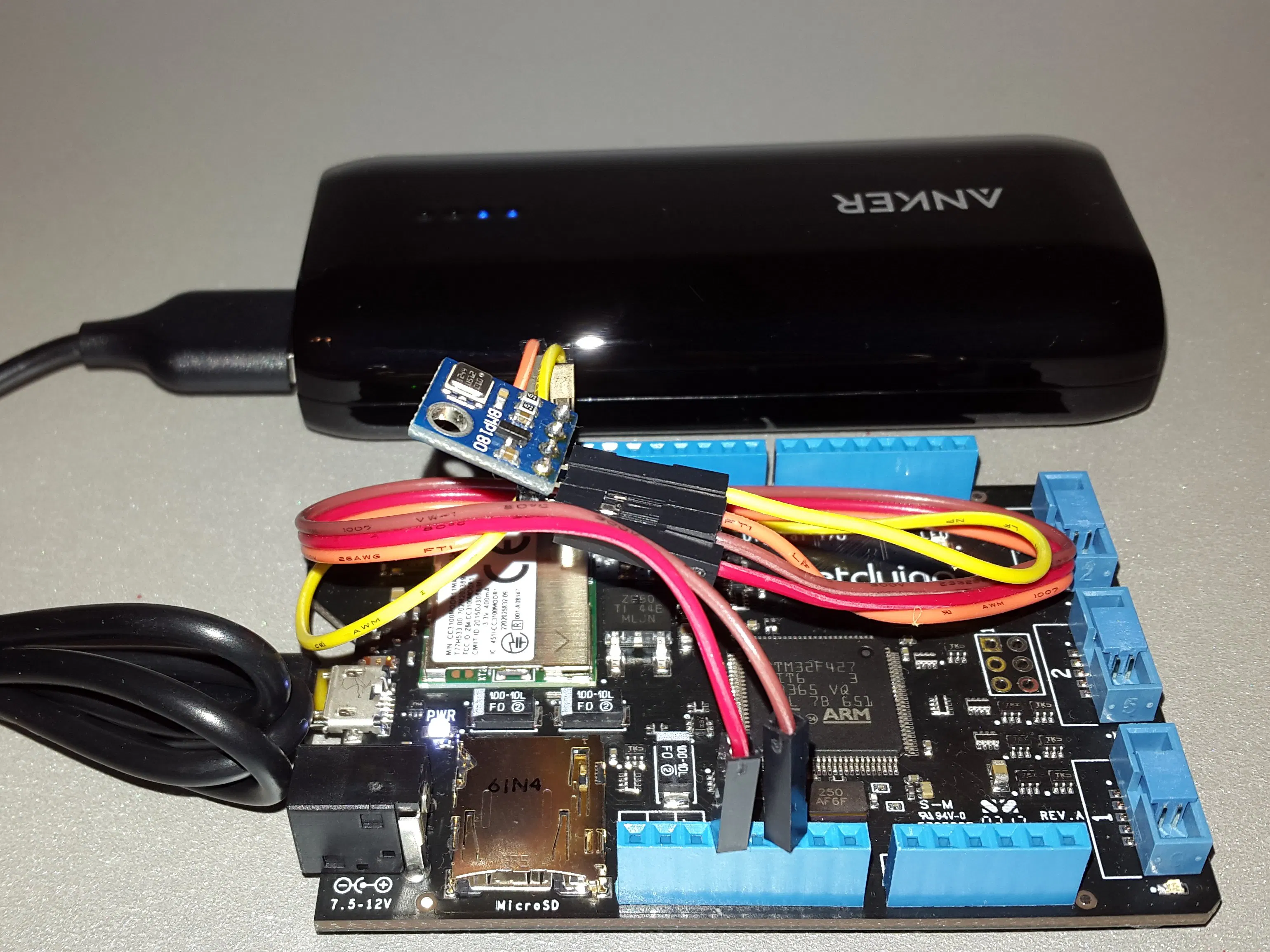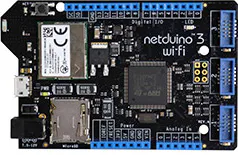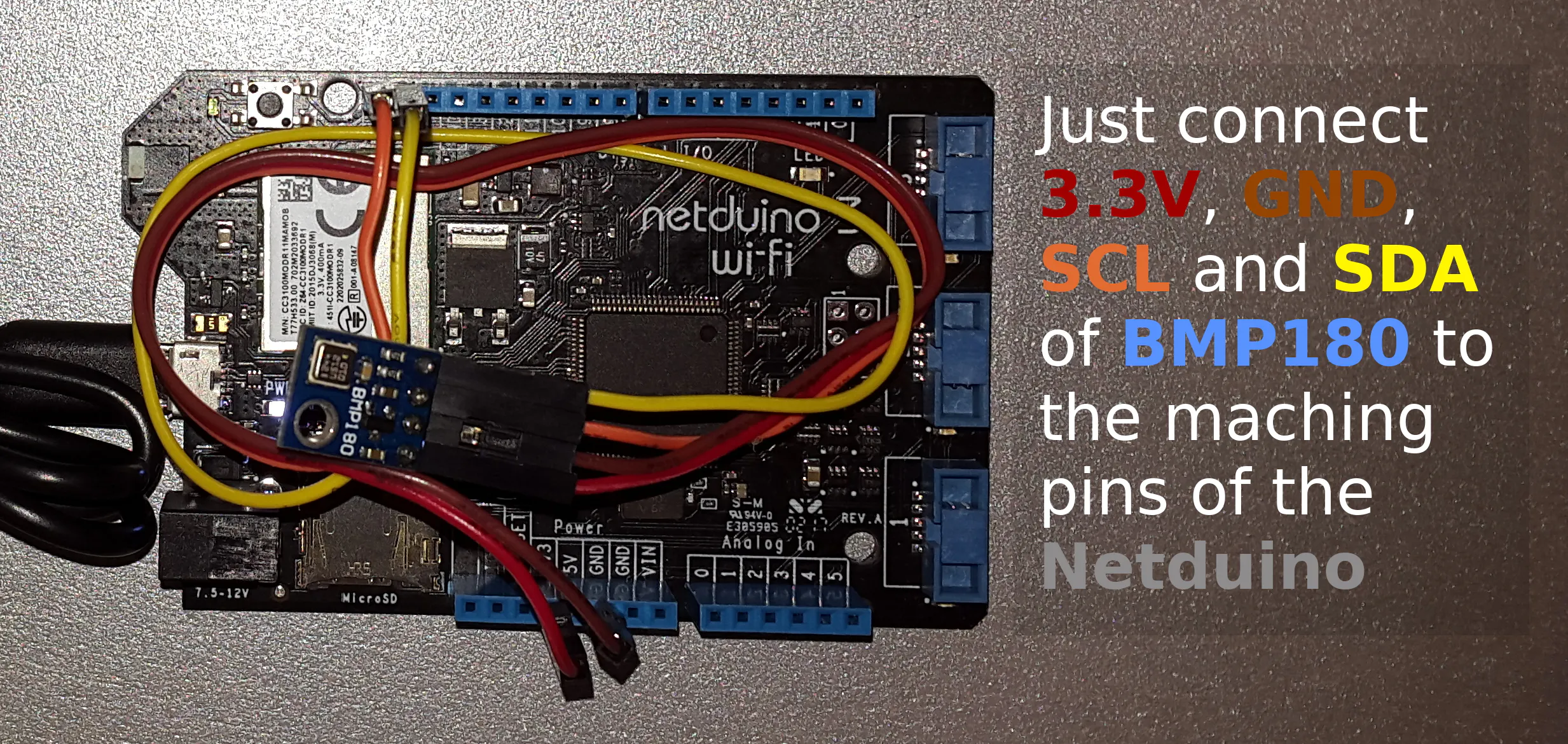# Battery Powered Weather & Air Quality Monitoring Station

A web-enabled weather station implemented with Netduino 3 WiFi and a BMP180 sensor.

BeginnerFull instructions provided8 hours1,267## Things used in this project

### Hardware componentsWilderness Labs Netduino3 WiFi
×1
 Bosch BMP180 barometric sensor
×1
 Power Bank any micro-USB power bank will do
×1

### Software apps and online servicesMicrosoft Visual Studio 2015

## Schematics

### Schematics## Code

### Weather Station - Source Code

C#
```using Microsoft.SPOT;
using Microsoft.SPOT.Hardware;
using Microsoft.SPOT.Net.NetworkInformation;
using SecretLabs.NETMF.Hardware.Netduino;
using System;
using System.IO;
using System.Net;
using System.Net.Sockets;
using System.Text;

using Math = System.Math;

{
public class Program
{
public static void Main()
{
OutputPort led = new OutputPort(Pins.ONBOARD_LED, false);

App app = new App();
app.Run();

BMP180 bmp180 = new BMP180();
bmp180.begin();

Socket serverSocket = new Socket(AddressFamily.InterNetwork, SocketType.Stream, ProtocolType.Tcp);
IPEndPoint listenerEndPoint = new IPEndPoint(IPAddress.Any, 80);
serverSocket.Bind(listenerEndPoint);
serverSocket.Listen(1);

Debug.Print("Waiting for HTTP connections (port 80)");
while (true)
{
var clientSocket = serverSocket.Accept();

led.Write(true);

Debug.Print("Got HTTP connection.");

double temp, pressure;
unsafe
{
int delay = bmp180.startTemperature();
bmp180.getTemperature(&temp);

delay = bmp180.startPressure(3);
bmp180.getPressure(&pressure, temp);
}

double normalizedPressure = bmp180.sealevel(pressure, 350 /* meters */);

Debug.Print("Temp: " + temp);
Debug.Print("Absolute Pressure: " + pressure);
Debug.Print("Normalized (sea level) Pressure: " + normalizedPressure);

string response = "{\n"
+ "\t\"temperature\": " + temp + ",\n"
+ "\t\"absolute pressure\": " + pressure + ",\n"
+ "\t\"normalized (sea level) pressure\": " + normalizedPressure + "\n"
+ "}";
string header = "HTTP/1.0 200 OK\r\nContent-Type: text; charset=utf-8\r\nContent-Length: " + response.Length.ToString() + "\r\nConnection: close\r\n\r\n";
clientSocket.Send(Encoding.UTF8.GetBytes(response), response.Length, SocketFlags.None);

Debug.Print("Sent response: " + response);
led.Write(false);
}
}
}

public class App
{
NetworkInterface[] _interfaces;

public bool IsRunning { get; set; }

public void Run()
{
this.IsRunning = true;
bool goodToGo = InitializeNetwork();

//if (goodToGo)
//{
//}

this.IsRunning = false;
}

protected bool InitializeNetwork()
{
if (Microsoft.SPOT.Hardware.SystemInfo.SystemID.SKU == 3)
{
Debug.Print("Wireless tests run only on Device");
return false;
}

Debug.Print("Getting all the network interfaces.");
_interfaces = NetworkInterface.GetAllNetworkInterfaces();

// debug output
ListNetworkInterfaces();

// loop through each network interface
foreach (var net in _interfaces)
{

// debug out
ListNetworkInfo(net);

switch (net.NetworkInterfaceType)
{
case (NetworkInterfaceType.Ethernet):
Debug.Print("Found Ethernet Interface");
break;
case (NetworkInterfaceType.Wireless80211):
Debug.Print("Found 802.11 WiFi Interface");
break;
case (NetworkInterfaceType.Unknown):
Debug.Print("Found Unknown Interface");
break;
}

// check for an IP address, try to get one if it's empty
}

// if we got here, should be false.
return false;
}

protected void MakeWebRequest(string url)
{
var httpWebRequest = (HttpWebRequest)WebRequest.Create(url);
httpWebRequest.Method = "GET";

var httpResponse = (HttpWebResponse)httpWebRequest.GetResponse();
{
Debug.Print("this is what we got from " + url + ": " + result);
}
}

{
int timeout = 10000; // timeout, in milliseconds to wait for an IP. 10,000 = 10 seconds

// check to see if the IP address is empty (0.0.0.0). IPAddress.Any is 0.0.0.0.
{

if (net.IsDhcpEnabled)
{
Debug.Print("DHCP is enabled, attempting to get an IP Address");

// ask for an IP address from DHCP [note this is a static, not sure which network interface it would act on]
int sleepInterval = 10;
int maxIntervalCount = timeout / sleepInterval;
int count = 0;
{
Debug.Print("Sleep while obtaining an IP");
count++;
};

// if we got here, we either timed out or got an address, so let's find out.
{
Debug.Print("Failed to get an IP Address in the alotted time.");
return false;
}

return true;

//NOTE: this does not work, even though it's on the actual network device. [shrug]
// try to renew the DHCP lease and get a new IP Address
//}

}
else
{
Debug.Print("DHCP is not enabled, and no IP address is configured, bailing out.");
return false;
}
}
else
{
return true;
}

}

protected void ListNetworkInterfaces()
{
foreach (var net in _interfaces)
{
switch (net.NetworkInterfaceType)
{
case (NetworkInterfaceType.Ethernet):
Debug.Print("Found Ethernet Interface");
break;
case (NetworkInterfaceType.Wireless80211):
Debug.Print("Found 802.11 WiFi Interface");
break;
case (NetworkInterfaceType.Unknown):
Debug.Print("Found Unknown Interface");
break;

}
}
}

protected void ListNetworkInfo(NetworkInterface net)
{
Debug.Print("DHCP enabled: " + net.IsDhcpEnabled.ToString());
Debug.Print("Dynamic DNS enabled: " + net.IsDynamicDnsEnabled.ToString());

if (net is Wireless80211)
{
var wifi = net as Wireless80211;
Debug.Print("SSID:" + wifi.Ssid.ToString());
}

}

private static string BytesToHexString(byte[] bytes)
{
string hexString = string.Empty;

// Create a character array for hexidecimal conversion.
const string hexChars = "0123456789ABCDEF";

// Loop through the bytes.
for (byte b = 0; b < bytes.Length; b++)
{
if (b > 0)
hexString += "-";

// Grab the top 4 bits and append the hex equivalent to the return string.
hexString += hexChars[bytes[b] >> 4];

// Mask off the upper 4 bits to get the rest of it.
hexString += hexChars[bytes[b] & 0x0F];
}

return hexString;
}
}

public unsafe class BMP180
{

short AC1, AC2, AC3, VB1, VB2, MB, MC, MD;
ushort AC4, AC5, AC6;
double c5, c6, mc, md, x0, x1, x2, y0, y1, y2, p0, p1, p2;
char _error;

I2CDevice i2cDevice;

const byte BMP180_REG_CONTROL = 0xF4;
const byte BMP180_REG_RESULT = 0xF6;
const byte BMP180_COMMAND_TEMPERATURE = 0x2E;
const byte BMP180_COMMAND_PRESSURE0 = 0x34;
const byte BMP180_COMMAND_PRESSURE1 = 0x74;
const byte BMP180_COMMAND_PRESSURE2 = 0xB4;
const byte BMP180_COMMAND_PRESSURE3 = 0xF4;

public bool begin()
// Initialize library for subsequent pressure measurements
{
double c3, c4, b1;

// Start up the Arduino's "wire" (I2C) library:

i2cDevice = new I2CDevice(new I2CDevice.Configuration(BMP180_ADDR, 50));

//Wire.begin();

// The BMP180 includes factory calibration data stored on the device.
// Each device has different numbers, these must be retrieved and
// used in the calculations when taking pressure measurements.

// Retrieve calibration data from device:
fixed (short* ac1 = &AC1, ac2 = &AC2, ac3 = &AC3, vb1 = &VB1, vb2 = &VB2, mb = &MB, mcc = &MC, mdd = &MD)
{
fixed (ushort* ac4 = &AC4, ac5 = &AC5, ac6 = &AC6)
{
{

// If you need to check your math using known numbers,
// you can uncomment one of these examples.
// (The correct results are commented in the below functions.)

// Example from Bosch datasheet
// AC1 = 408; AC2 = -72; AC3 = -14383; AC4 = 32741; AC5 = 32757; AC6 = 23153;
// B1 = 6190; B2 = 4; MB = -32768; MC = -8711; MD = 2868;

// Example from http://wmrx00.sourceforge.net/Arduino/BMP180-Calcs.pdf
// AC1 = 7911; AC2 = -934; AC3 = -14306; AC4 = 31567; AC5 = 25671; AC6 = 18974;
// VB1 = 5498; VB2 = 46; MB = -32768; MC = -11075; MD = 2432;

/*
Serial.print("AC1: "); Serial.println(AC1);
Serial.print("AC2: "); Serial.println(AC2);
Serial.print("AC3: "); Serial.println(AC3);
Serial.print("AC4: "); Serial.println(AC4);
Serial.print("AC5: "); Serial.println(AC5);
Serial.print("AC6: "); Serial.println(AC6);
Serial.print("VB1: "); Serial.println(VB1);
Serial.print("VB2: "); Serial.println(VB2);
Serial.print("MB: "); Serial.println(MB);
Serial.print("MC: "); Serial.println(MC);
Serial.print("MD: "); Serial.println(MD);
*/

// Compute floating-point polynominals:

c3 = 160.0 * Math.Pow(2, -15) * AC3;
c4 = Math.Pow(10, -3) * Math.Pow(2, -15) * AC4;
b1 = Math.Pow(160, 2) * Math.Pow(2, -30) * VB1;
c5 = (Math.Pow(2, -15) / 160) * AC5;
c6 = AC6;
mc = (Math.Pow(2, 11) / Math.Pow(160, 2)) * MC;
md = MD / 160.0;
x0 = AC1;
x1 = 160.0 * Math.Pow(2, -13) * AC2;
x2 = Math.Pow(160, 2) * Math.Pow(2, -25) * VB2;
y0 = c4 * Math.Pow(2, 15);
y1 = c4 * c3;
y2 = c4 * b1;
p0 = (3791.0 - 8.0) / 1600.0;
p1 = 1.0 - 7357.0 * Math.Pow(2, -20);
p2 = 3038.0 * 100.0 * Math.Pow(2, -36);

/*
Serial.println();
Serial.print("c3: "); Serial.println(c3);
Serial.print("c4: "); Serial.println(c4);
Serial.print("c5: "); Serial.println(c5);
Serial.print("c6: "); Serial.println(c6);
Serial.print("b1: "); Serial.println(b1);
Serial.print("mc: "); Serial.println(mc);
Serial.print("md: "); Serial.println(md);
Serial.print("x0: "); Serial.println(x0);
Serial.print("x1: "); Serial.println(x1);
Serial.print("x2: "); Serial.println(x2);
Serial.print("y0: "); Serial.println(y0);
Serial.print("y1: "); Serial.println(y1);
Serial.print("y2: "); Serial.println(y2);
Serial.print("p0: "); Serial.println(p0);
Serial.print("p1: "); Serial.println(p1);
Serial.print("p2: "); Serial.println(p2);
*/

// Success!
return true;
}
else
{
return false;
}
}
}
}

// Read a signed integer (two bytes) from device
// value: external variable to store data (function modifies value)
{
byte[] data = new byte;

{
*value = (short)(((short)data << 8) | (short)data);
//if (*value & 0x8000) *value |= 0xFFFF0000; // sign extend if negative
return true;
}
*value = 0;
return false;
}

// Read an unsigned integer (two bytes) from device
// value: external variable to store data (function modifies value)
{
byte[] data = new byte;

{
*value = (ushort)(((ushort)data << 8) | (ushort)data);
return true;
}
*value = 0;
return false;
}

// Read an array of bytes from device
// values: external array to hold data. Put starting register in values.
// length: number of bytes to read
{
char x;

int bytesWritten = i2cDevice.Execute(new I2CDevice.I2CTransaction[] {
I2CDevice.CreateWriteTransaction(new byte[] { values })
}, 100);

if (bytesWritten > 0)
{
int bytesRead = i2cDevice.Execute(new I2CDevice.I2CTransaction[] {
}, 100);

return true;
}
return false;
}

bool writeBytes(byte[] values, byte length)
// Write an array of bytes to device
// values: external array of data to write. Put starting register in values.
// length: number of bytes to write
{
char x;

int bytesWritten = i2cDevice.Execute(new I2CDevice.I2CTransaction[] {
I2CDevice.CreateWriteTransaction(values)
}, 100);

if (bytesWritten > 0)
return true;
else
return false;
}

public int startTemperature()
// Will return delay in ms to wait, or 0 if I2C error
{
byte[] data = new byte;
bool result;

data = BMP180_REG_CONTROL;
data = BMP180_COMMAND_TEMPERATURE;
result = writeBytes(data, 2);
if (result) // good write?
return (5); // return the delay in ms (rounded up) to wait before retrieving data
else
return (0); // or return 0 if there was a problem communicating with the BMP
}

public bool getTemperature(double* T)
// Retrieve a previously-started temperature reading.
// Requires begin() to be called once prior to retrieve calibration parameters.
// Requires startTemperature() to have been called prior and sufficient time elapsed.
// T: external variable to hold result.
// Returns 1 if successful, 0 if I2C error.
{
byte[] data = new byte;
bool result;
double tu, a;

data = BMP180_REG_RESULT;

if (result) // good read, calculate temperature
{
tu = (data * 256.0) + data;

//example from Bosch datasheet
//tu = 27898;

//example from http://wmrx00.sourceforge.net/Arduino/BMP085-Calcs.pdf
//tu = 0x69EC;

a = c5 * (tu - c6);
*T = a + (mc / (a + md));

/*
Serial.println();
Serial.print("tu: "); Serial.println(tu);
Serial.print("a: "); Serial.println(a);
Serial.print("T: "); Serial.println(*T);
*/
}
return (result);
}

public byte startPressure(byte oversampling)
// Oversampling: 0 to 3, higher numbers are slower, higher-res outputs.
// Will return delay in ms to wait, or 0 if I2C error.
{
byte[] data = new byte;
bool result;
byte delay;

data = BMP180_REG_CONTROL;

switch (oversampling)
{
case 0:
data = BMP180_COMMAND_PRESSURE0;
delay = 5;
break;
case 1:
data = BMP180_COMMAND_PRESSURE1;
delay = 8;
break;
case 2:
data = BMP180_COMMAND_PRESSURE2;
delay = 14;
break;
case 3:
data = BMP180_COMMAND_PRESSURE3;
delay = 26;
break;
default:
data = BMP180_COMMAND_PRESSURE0;
delay = 5;
break;
}
result = writeBytes(data, 2);
if (result) // good write?
return (delay); // return the delay in ms (rounded up) to wait before retrieving data
else
return (0); // or return 0 if there was a problem communicating with the BMP
}

public bool getPressure(double* P, double T)
// Retrieve a previously started pressure reading, calculate abolute pressure in mbars.
// Requires begin() to be called once prior to retrieve calibration parameters.
// Requires startPressure() to have been called prior and sufficient time elapsed.
// Requires recent temperature reading to accurately calculate pressure.

// P: external variable to hold pressure.
// T: previously-calculated temperature.
// Returns 1 for success, 0 for I2C error.

// Note that calculated pressure value is absolute mbars, to compensate for altitude call sealevel().
{
byte[] data = new byte;
bool result;
double pu, s, x, y, z;

data = BMP180_REG_RESULT;

if (result) // good read, calculate pressure
{
pu = (data * 256.0) + data + (data / 256.0);

//example from Bosch datasheet
//pu = 23843;

//example from http://wmrx00.sourceforge.net/Arduino/BMP085-Calcs.pdf, pu = 0x982FC0;
//pu = (0x98 * 256.0) + 0x2F + (0xC0/256.0);

s = T - 25.0;
x = (x2 * Math.Pow(s, 2)) + (x1 * s) + x0;
y = (y2 * Math.Pow(s, 2)) + (y1 * s) + y0;
z = (pu - x) / y;
*P = (p2 * Math.Pow(z, 2)) + (p1 * z) + p0;

/*
Serial.println();
Serial.print("pu: "); Serial.println(pu);
Serial.print("T: "); Serial.println(*T);
Serial.print("s: "); Serial.println(s);
Serial.print("x: "); Serial.println(x);
Serial.print("y: "); Serial.println(y);
Serial.print("z: "); Serial.println(z);
Serial.print("P: "); Serial.println(*P);
*/
}
return (result);
}

public double sealevel(double P, double A)
// Given a pressure P (mb) taken at a specific altitude (meters),
// return the equivalent pressure (mb) at sea level.
// This produces pressure readings that can be used for weather measurements.
{
return (P / Math.Pow(1 - (A / 44330.0), 5.255));
}

public double altitude(double P, double P0)
// Given a pressure measurement P (mb) and the pressure at a baseline P0 (mb),
// return altitude (meters) above baseline.
{
return (44330.0 * (1 - Math.Pow(P / P0, 1 / 5.255)));
}

public char getError()
// If any library command fails, you can retrieve an extended
// error code using this command. Errors are from the wire library:
// 0 = Success
// 1 = Data too long to fit in transmit buffer
// 3 = Received NACK on transmit of data
// 4 = Other error
{
return (_error);
}
}
}
```

## Credits

### Attila Tőkés

32 projects • 172 followers
Software Engineer experimenting with hardware projects involving IoT, Computer Vision, ML & AI, FPGA, Crypto and other related technologies.